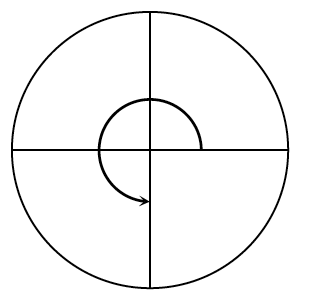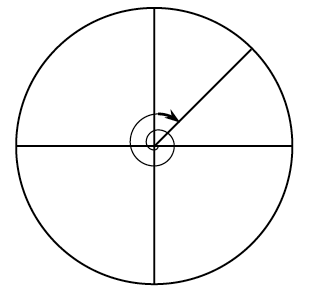### Home > PC > Chapter 3 > Lesson 3.3.2 > Problem3-129

3-129.

​Below are two of the special angles that are used in the unit circle. Identify the radian measure for each angle shown. Remember, counterclockwise angles are positive and clockwise angles are negative.

1.A full circle is an angle of $2\pi$ radians. What is 3/4s of that?

1.Divide the circle into eight equal pieces. Then remember that four of those pieces are equivalent to $\pi$ radians.